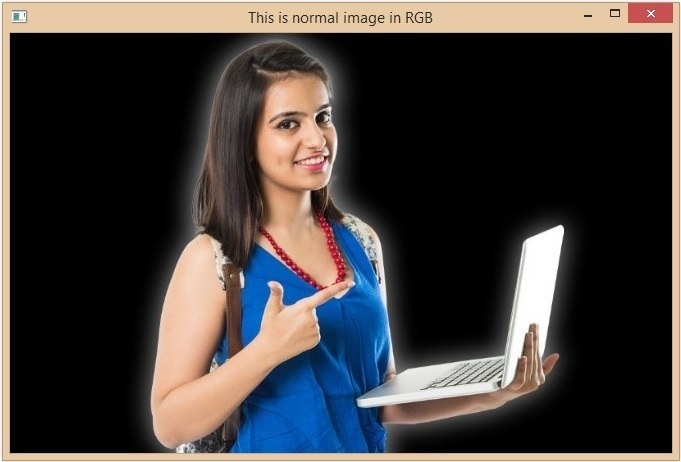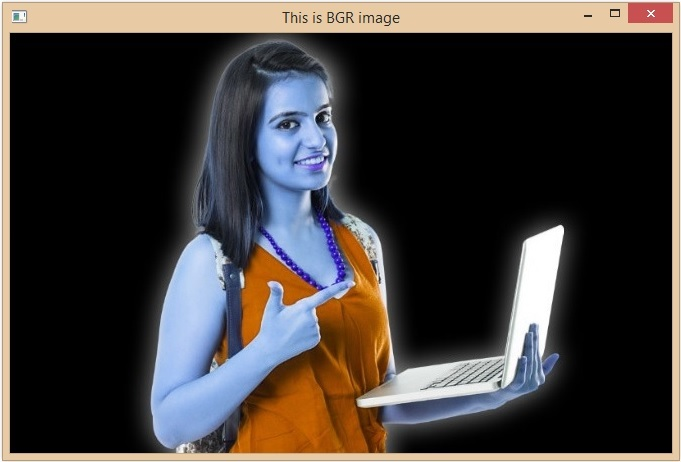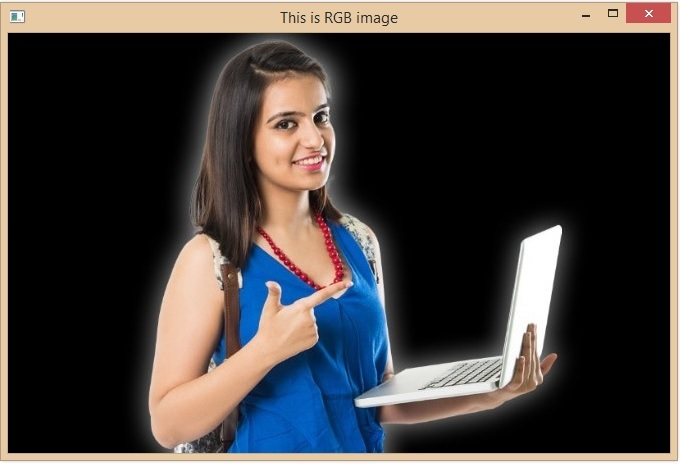# Convert BGR and RGB with Python and OpenCV

OpenCV is a library of programming functions primarily for real-time computer vision. The library is cross-platform and licensed as free and open source software under the Apache License. It is written in C++ and has Python bindings that make it easy to use in Python applications.

In this article we are going to learn how to Convert BGR and RGB with python and OpenCV. Before proceeding further first of all let us understand BGR and RGB.

## What are BGR and RGB

RGB and BGR are both color models used to represent colors in digital images. However, the main difference between these two color models is the order in which the color channels are arranged.

• In the RGB color model, the colors are represented by the Red, Green, and Blue color channels. The red channel shows information about the amount of red color similarly for the blue and green channel. On combining these information, we get the whole image.

• Whereas in the BGR model which is also called OpenCV model the color channels are a reverse of the RGB model. But the particular light contains information about the intensity of that particular light.

The layout of the Red, Green, and Blue subpixels is the primary distinction between RGB and BGR. Although BGR is basically in reverse of RGB, there is no difference in the vibrancy and accuracy of the colours. While some BGR screens, like the Gigabyte M27Q, even give a colour accuracy score that's suitable for editing work, they look great for games and movies.

Let us see steps how we can perform custom seed-based contour detection using Python and OpenCV

### Step 1: Importing the Required Libraries

To convert images between the BGR and RGB color spaces, we need to import the required libraries. We will be using the following libraries −

• OpenCV − To read and manipulate images.

• Matplotlib − To display images.

import cv2
import matplotlib.pyplot as plt


### Step 2: Reading the Image

The first step in converting an image between the BGR and RGB color spaces is to read the image. We can use the cv2.imread function to read an image from a file.

In this snippet, we have used the cv2.cvtColor function to convert the BGR image to RGB before displaying it using the matplotlib library.

# Read the image in BGR color space

# Display the image
cv2.imshow('this is normal image in RGB', img)
cv2.waitKey(0)
cv2.destroyAllWindows()


### Step 3: Converting from RGB to BGR

To convert an image from the BGR color space to the RGB color space, we simply need to use the cv2.cvtColor function and pass the BGR image and the cv2.COLOR_BGR2RGB flag as inputs.

In this snippet, we have converted the BGR image to RGB and displayed it using the matplotlib library.

# Convert the image from RGB to BGR
bgr_img = cv2.cvtColor(img, cv2.COLOR_RGB2BGR)

# Display the BGR image
cv2.imshow('this is BGR image', bgr_image)
cv2.waitKey(0)
cv2.destroyAllWindows()


### Step 4: Converting from BGR to RGB

To convert an image from the RGB color space to the BGR color space, we can use the same cv2.cvtColor function and pass the RGB image and the cv2.COLOR_RGB2BGR flag as inputs.

In this snippet, we have converted the RGB image to BGR and displayed it using the matplotlib library.

# Convert the image from BGR to RGB
rgb_img = cv2.cvtColor(img, cv2.COLOR_BGR2RGB)

# Display the RGB image
cv2.imshow('this is RGB image', rgb_img)
cv2.waitKey(0)
cv2.destroyAllWindows()


## Example

Following is the complete example –

import cv2
import matplotlib.pyplot as plt

# Read the image in BGR color space

# Display the image
cv2.imshow('This is normal image in RGB', img)
cv2.waitKey(0)
cv2.destroyAllWindows()

# Convert the image from RGB to BGR
bgr_img = cv2.cvtColor(img, cv2.COLOR_RGB2BGR)

# Display the BGR image
cv2.imshow('This is BGR image', bgr_img)
cv2.waitKey(0)
cv2.destroyAllWindows()

# Convert the image from BGR to RGB
rgb_img = cv2.cvtColor(img, cv2.COLOR_BGR2RGB)

# Display the RGB image
cv2.imshow('This is RGB image', rgb_img)
cv2.waitKey(0)
cv2.destroyAllWindows()


## Output

Original Image −RGB to BGR −Back to RGB −## Conclusion

In this article, we have explained how to convert images from BGR to RGB and vice versa. We explored the RGB and BGR color spaces. We have gone through all the steps to convert an image from an RGB image to a BGR image. By following all the steps in this article, you can easily convert an image from one color space to another using the OpenCV library of python language.

Updated on: 20-Apr-2023

4K+ Views